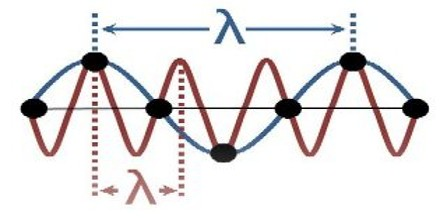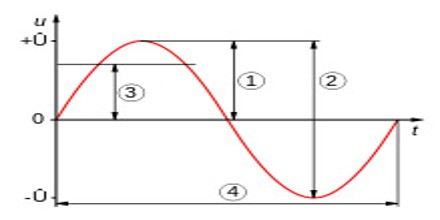Amplitude

Definition

Amplitude is an ocean wave is the maximum height of the wave crest above the level of calm water, or the maximum depth of the wave trough below the level of calm water. The amplitude of a pendulum swinging through an angle of 90° is 45°. It is equal to one-half the length of the vibration path. The amplitude of a pendulum is thus one-half the distance that the bob traverses in moving from one side to the other. Waves are generated by vibrating sources, their amplitude being proportional to the amplitude of the source.There are various definitions of amplitude, which are all functions of the magnitude of the difference between the variable’s extreme values. Amplitude is the maximum displacement of points on a wave, which we can think of as the degree or intensity of change. This maximum displacement is measured from the equilibrium position.

For a transverse wave, such as the wave on a plucked string, amplitude is measured by the maximum displacement of any point on the string from its position when the string is at rest. For a longitudinal wave, such as a sound wave, amplitude is measured by the maximum displacement of a particle from its position of equilibrium. When the amplitude of a wave steadily decreases because its energy is being lost, it is said to be damped.

Amplitude is the most widely used measure of orbital wobble in astronomy and the measurement of small radial velocity semi-amplitudes of nearby stars is important in the search for exoplanets.Frequency and Units of Amplitude

The frequency is the number of wave cycles passing a point per unit time. Stated another way, it is the number of oscillations per second in the wave. A higher frequency means a shorter wavelength, and a lower frequency means a longer wavelength.The units of the amplitude depend on the type of wave, but are always in the same units as the oscillating variable. A more general representation of the wave equation is more complex, but the role of amplitude remains analogous to this simple case. The logarithm of the amplitude squared is usually quoted in dB, so null amplitude corresponds to −∞ dB. Loudness is related to amplitude and intensity and is one of the most salient qualities of a sound, although in general sounds can be recognized independently of amplitude. The square of the amplitude is proportional to the intensity of the wave.

Although wavelength and frequency are related, amplitude and frequency are independent features of a wave. Both parameters can be changed, but changing only one does not affect the other. Changing frequency does not alter the amplitude of the wave, and changing the amplitude does not alter the frequency. The amplitude as defined above is a constant and the wave is said to be wavelength continuous.

Reference: britannica.com, dictionary.com, study.com, wikipedia.